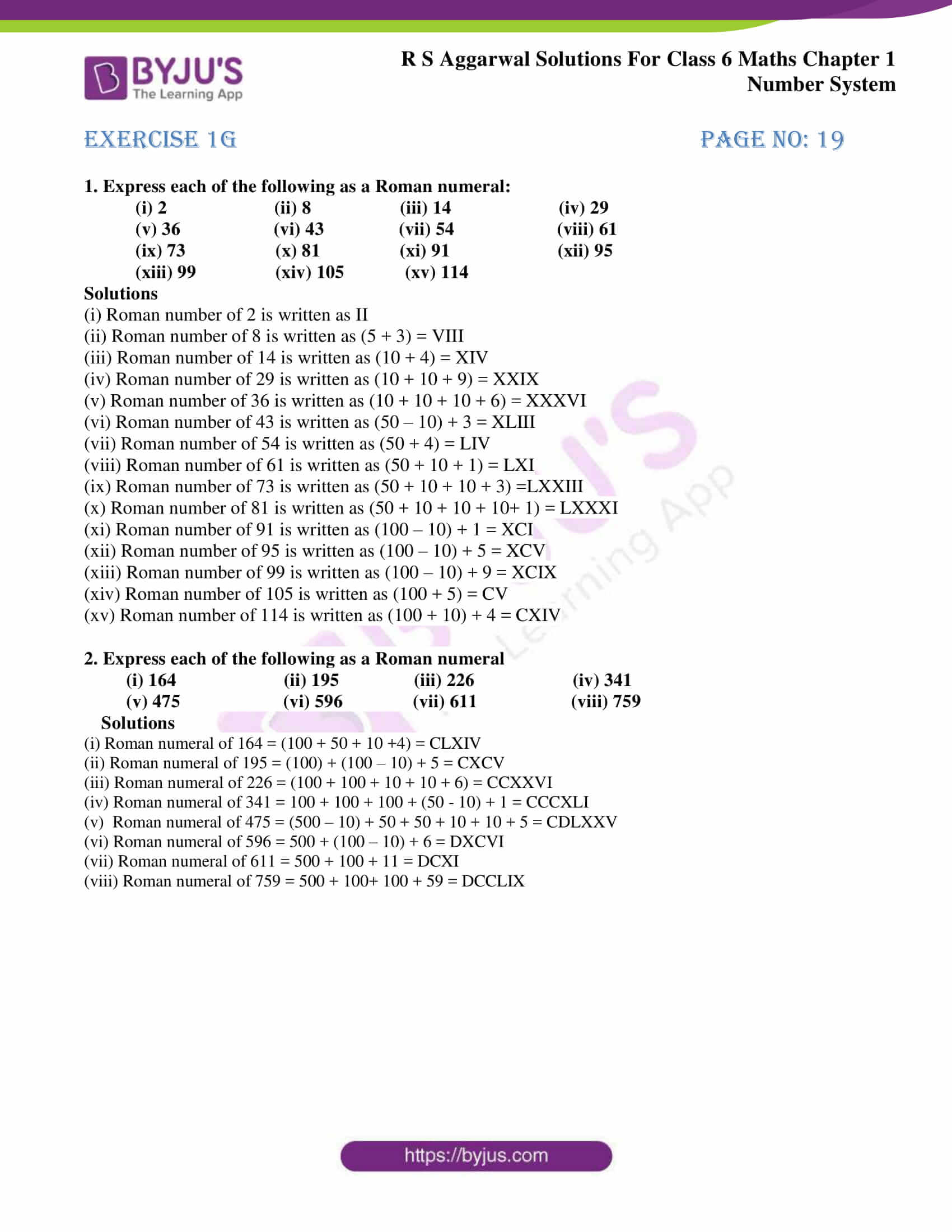# RS Aggarwal Solutions for Class 6 Chapter 1 Number System Exercise 1G

RS Aggarwal Solutions for Class 6, Chapter 1, Number System Exercise 1G available in simple PDF in this page. This exercise mainly helps students to learn about the concept of Roman numerals with the help of solutions designed by experts. Students who practice RS Aggarwal Solutions for Class 6 Chapter 1 Number System understand the chapter in detail with ease.

The solutions are designed by experts with explanations which match the understanding capacity of students. Students are advised to practice RS Aggarwal Solutions for Class 6 Chapter 1 Number System as they provide the solutions comprehensively.

## Download PDF of RS Aggarwal Solutions for Class 6 Chapter 1 Number System Exercise 1G### Access answers to Maths RS Aggarwal Solutions for Class 6 Chapter 1 Number System Exercise 1G

1. Express each of the following as a Roman numeral:

(i) 2 (ii) 8 (iii) 14 (iv) 29

(v) 36 (vi) 43 (vii) 54 (viii) 61

(ix) 73 (x) 81 (xi) 91 (xii) 95

(xiii) 99 (xiv) 105 (xv) 114

Solutions

(i) Roman number of 2 is written as II

(ii) Roman number of 8 is written as (5 + 3) = VIII

(iii) Roman number of 14 is written as (10 + 4) = XIV

(iv) Roman number of 29 is written as (10 + 10 + 9) = XXIX

(v) Roman number of 36 is written as (10 + 10 + 10 + 6) = XXXVI

(vi) Roman number of 43 is written as (50 – 10) + 3 = XLIII

(vii) Roman number of 54 is written as (50 + 4) = LIV

(viii) Roman number of 61 is written as (50 + 10 + 1) = LXI

(ix) Roman number of 73 is written as (50 + 10 + 10 + 3) =LXXIII

(x) Roman number of 81 is written as (50 + 10 + 10 + 10+ 1) = LXXXI

(xi) Roman number of 91 is written as (100 – 10) + 1 = XCI

(xii) Roman number of 95 is written as (100 – 10) + 5 = XCV

(xiii) Roman number of 99 is written as (100 – 10) + 9 = XCIX

(xiv) Roman number of 105 is written as (100 + 5) = CV

(xv) Roman number of 114 is written as (100 + 10) + 4 = CXIV

2. Express each of the following as a Roman numeral

(i) 164 (ii) 195 (iii) 226 (iv) 341

(v) 475 (vi) 596 (vii) 611 (viii) 759

Solutions

(i) Roman numeral of 164 = (100 + 50 + 10 +4) = CLXIV

(ii) Roman numeral of 195 = (100) + (100 – 10) + 5 = CXCV

(iii) Roman numeral of 226 = (100 + 100 + 10 + 10 + 6) = CCXXVI

(iv) Roman numeral of 341 = 100 + 100 + 100 + (50 – 10) + 1 = CCCXLI

(v) Roman numeral of 475 = (500 – 10) + 50 + 50 + 10 + 10 + 5 = CDLXXV

(vi) Roman numeral of 596 = 500 + (100 – 10) + 6 = DXCVI

(vii) Roman numeral of 611 = 500 + 100 + 11 = DCXI

(viii) Roman numeral of 759 = 500 + 100+ 100 + 59 = DCCLIX

## RS Aggarwal Solutions for Class 6 Maths Chapter 1 Number System Exercise 1G

Exercise 1G of RS Aggarwal textbook for Class 6 Chapter 1 Number System explains the concept of Roman numerals. By regular practice of problems using RS Aggarwal solutions the students gain a better hold on the concept. Topics present in other exercises are as follows

• International system of numeration
• Comparison of numbers
• Word problems on number operations
• Estimation
• Estimating the products
• Estimating the quotients

RS Aggarwal Solutions helps students to solve the questions of RS Aggarwal textbook with ease as they give perfect solutions in PDF format. By continuously practising RS Aggarwal Solutions, students not only score high marks but also boost their confidence in Mathematics.